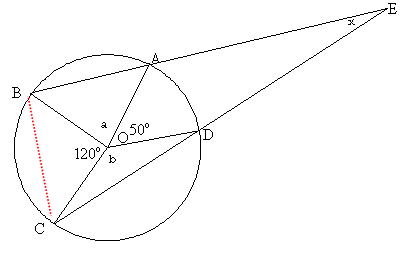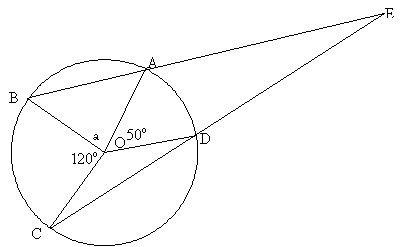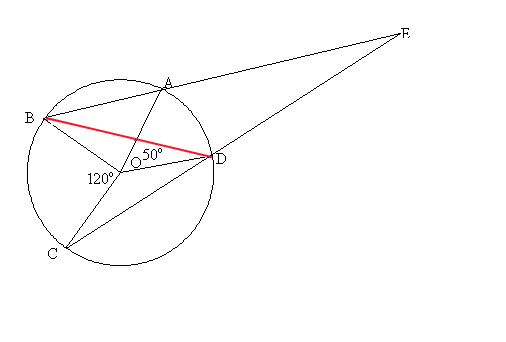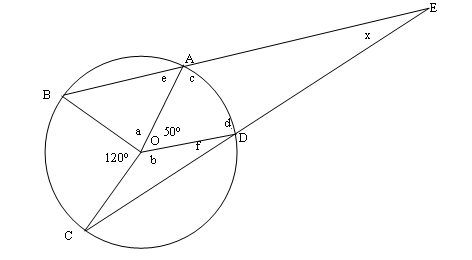#### You may also like### Diophantine N-tuples

Can you explain why a sequence of operations always gives you perfect squares?### DOTS Division

Take any pair of two digit numbers x=ab and y=cd where, without loss of generality, ab > cd . Form two 4 digit numbers r=abcd and s=cdab and calculate: {r^2 - s^2} /{x^2 - y^2}.### Sixational

The nth term of a sequence is given by the formula n^3 + 11n . Find the first four terms of the sequence given by this formula and the first term of the sequence which is bigger than one million. Prove that all terms of the sequence are divisible by 6.

# Lens Angle

##### Age 14 to 16 Challenge Level:

There were almost as many solutions to this problem as solutions sent in! I have included four versions below. It is good to see that there is more than one way to answer most NRICH problems. Well done to all of you.

Just one general comment - some of you assumed that AOC was a diameter and, although this led to the same answer, the solution you gave was therefore not correct.

The first solution is from Julia Collins from Langley Park School for Girls, Bromley.Since OC = OD, OCD is an isosceles triangle and angle OCD = angle ODC.
Angle OCD = $\frac{180 - b}{2} = 90 - \frac{b}{2}$
Similarly with triangles OCB (giving angles of $30$° for OBC and OCB) and OBA (giving angle OBA = $90 - \frac{a}{2}$).

Triangle BCE must have its angles adding up to 180°.
Therefore:

$(90 - \frac{a}{2}) + 30 + 30 + (90 - \frac{b}{2}) + x = 180$
$60 + x - \frac{a}{2} - \frac{b}{2} = 0$
$120 + 2x= a + b$

The angles around the centre of the circle must add up to $360^\circ$, so $a + b = 360 - 120 - 50 = 190^\circ$

So $120 + 2x = 190$
$2x = 70$
$x = 35^\circ$

The second solution is from Andrei Lazanu from School 205, Bucharest, RomaniaTriangles OAB and OCD are both isosceles, OA, OB, OC and OD being radii in the circle of centre O.

If a is the measure of angle AOB, the measure of angles OAB and OBA is: $${{{180^o}-a} \over 2} = 90^o - {a \over 2}$$ The measure of angle OAE is consequently: $${{180^o} - ({90^o} - {a \over 2})} = 90^o + {a \over 2}$$ Because the measure of an angle round a point is $360$°, the measure of angle COD is: $${360^o}- ({120^o} + {50^o} + a) = {190^o} -a$$ The base angles of the isosceles triangle BOD have the measure: $${{{180^o} - ({190^o} - a)} \over 2} = {{a \over2} - {5^o}}$$ The angle ODE has the measure: $${{180^o} - ({a \over 2} - {5^o})} = {{185^o} - {a \over 2}}$$ I use now the sum of angles in the quadrilateral OAED, that is $360$°, in order to determine the measure of angle DEA: $${360^o}- ( {50^o} + {185^o} - {a \over 2} + {90^o} + {a \over 2}) = {35^o}$$ Consequently, the measure of angle DEA is $35$°.

Guy David from Ransom Everglades Middle, Miami, Fl, USA sent this solutionThe measure of the mystery angle is $35$ degrees.

The inscribed angle in a circle is half the measure of the arc it intercepts.

First, I make chord BD. This creates an inscribed angle of measure $120^\circ /2 = 60^\circ$ at D. Which means that angle BDE is $120$o .

The chord also create an inscribed angle at B, of measure $50^\circ /2 = 25^\circ$.

We now have two angles of triangle EBD, that sum to $145$o . Therefore, the mystery angle is $180^\circ -145^\circ = 35^\circ$.

Anna Robinson, Caroline Swanson and Chloe Pickup from The City of London School for Girls$170 + a + b =360$
$a + b = 360 - 170$
$a + b = 190$

In triangles OBA and OBC

$a + b + 2e + 2f = 360$
Therefore $2e + 2f = 360- 190$
$2e + 2f =170$ (divide by $2$)
$e + f =85$ (*)

In OAED
$50 + c + d + x = 360$
$c + d + x = 310$ (**)

$e + d + f + c = 360$ (angles on straight lines)
$c + d = 275$ (from *)

$310 - 275 = 35$ from (** and above)

Therefore $x = 35$